International
Tables for
Crystallography
Volume F
Crystallography of biological macromolecules
Edited by M. G. Rossmann and E. Arnold

International Tables for Crystallography (2006). Vol. F, ch. 5.2, pp. 117-118   | 1 | 2 |

Section 5.2.4. Algebraic concepts

E. M. Westbrooka*

aMolecular Biology Consortium, Argonne, Illinois 60439, USA
Correspondence e-mail: westbrook@anl.gov

5.2.4. Algebraic concepts

| top | pdf |

Let V be the volume of one unit cell of the crystal. Let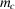be the total mass within one unit cell, and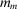,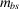and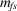be the masses, within one unit cell, of the macromolecule, bound solvent and free solvent, respectively. Let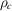,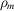,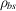and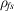, respectively, be the densities of a complete macromolecular crystal, its unsolvated macromolecule, its bound-solvent compartment and its free-solvent compartment. Let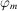,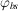and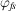, respectively, be the fractions of the crystal volume occupied by the unsolvated macromolecule, the bound solvent and the free solvent. By conservation of mass,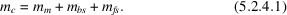The volume fractions must all add to unity: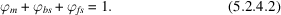The density of the crystal is the total mass divided by the unit-cell volume: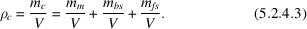The mass in each solvent compartment is the product of its density and the volume it occupies: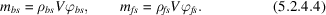The mass of the macromolecule in the cell can be defined either from its partial specific volume,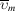, the unit-cell volume, V, and the molecules' volume fraction,, or from the molar weight, M, the number of molecular copies in the unit cell, n, and Avogadro's number,: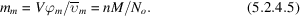Now (5.2.4.3) may be rewritten as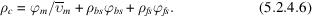Define a mean solvent density,: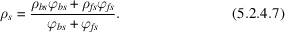This allows (5.2.4.6) to be rewritten as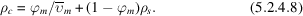Upon rearrangement, this gives expressions for the volume fraction of a macromolecule and for the molecular-packing number: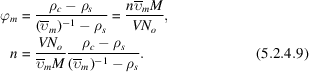In (5.2.4.9), all terms can be measured directly, except. The treatment ofwill be discussed in Section 5.2.7. (5.2.4.9) defines the total macromolecular mass in the unit cell,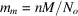, from a measurement of the crystal density. If M were known from the primary sequence of the molecule, this measurement determines the molecular-packing number, n, with considerable certainty. If the molar weight were not accurately known, it could be determined by measuring the crystal density.